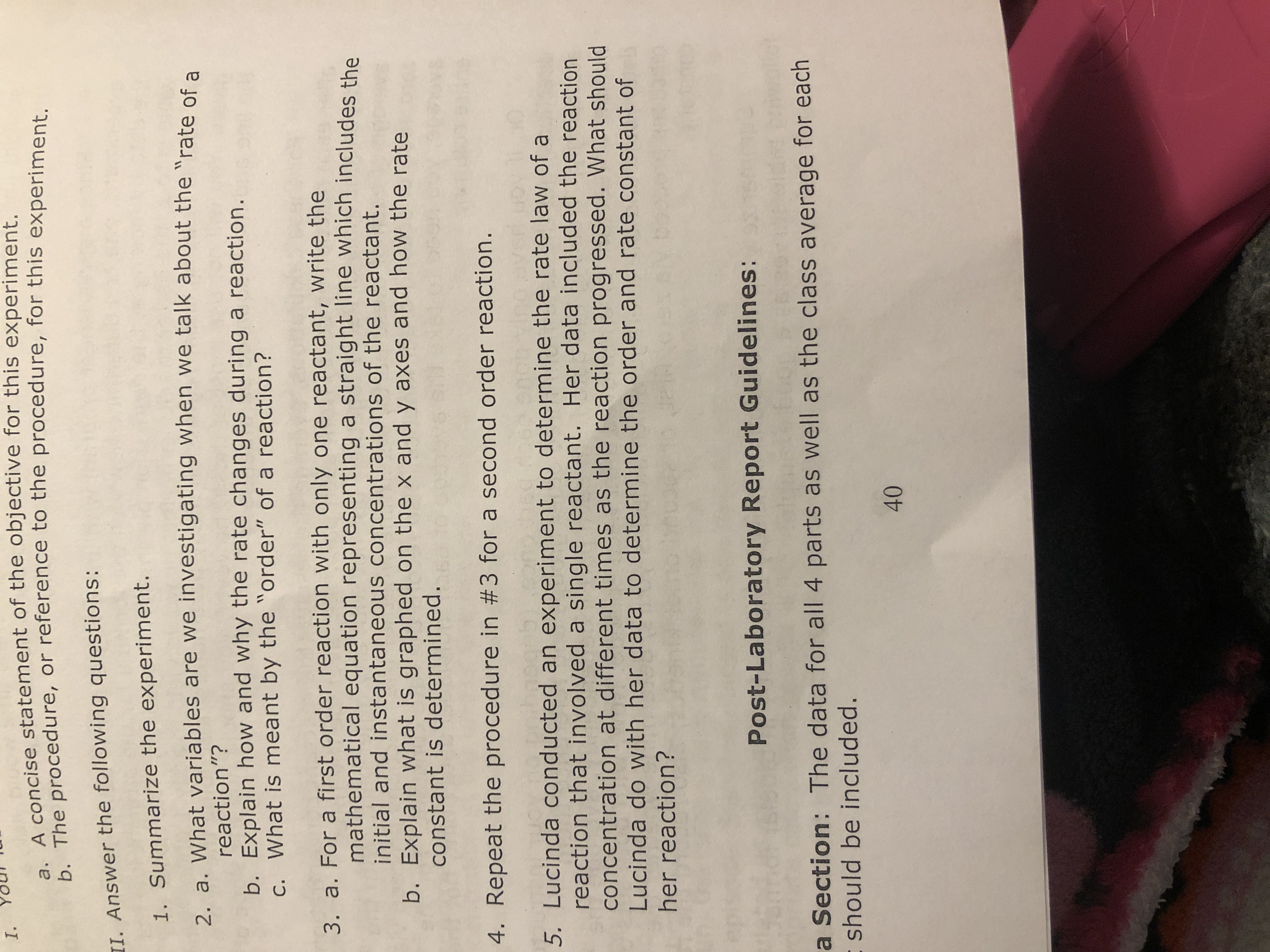# I.A concise statement of the objective for this experiment.b. The procedure, or reference to the procedure, for this experiment.II. Answer the following questions:1. Summarize the experiment.2 a. What variables are we investigating when we talk about the "rate of areaction"?b. Explain how and why the rate changes during a reaction.c. What is meant by the "order" of a reaction?3. a. For a first order reaction with only one reactant, write themathematical equation representing a straight line which includes theinitial and instantaneous concentrations of the reactant.b. Explain what is graphed on the x and y axes and how the rateconstant is determined.4. Repeat the procedure in #3 for a second order reaction.5. Lucinda conducted an experiment to determine the rate law of areaction that involved a single reactant. Her data included the reactionconcentration at different times as the reaction progressed. What shouldLucinda do with her data to determine the order and rate constant ofher reaction?Post-Laboratory Report Guidelines:a Section: The data for all 4 parts as well as the class average for each- should be included.40

Question
1 views

I need help with 3,4, and 5.help_outlineImage TranscriptioncloseI. A concise statement of the objective for this experiment. b. The procedure, or reference to the procedure, for this experiment. II. Answer the following questions: 1. Summarize the experiment. 2 a. What variables are we investigating when we talk about the "rate of a reaction"? b. Explain how and why the rate changes during a reaction. c. What is meant by the "order" of a reaction? 3. a. For a first order reaction with only one reactant, write the mathematical equation representing a straight line which includes the initial and instantaneous concentrations of the reactant. b. Explain what is graphed on the x and y axes and how the rate constant is determined. 4. Repeat the procedure in #3 for a second order reaction. 5. Lucinda conducted an experiment to determine the rate law of a reaction that involved a single reactant. Her data included the reaction concentration at different times as the reaction progressed. What should Lucinda do with her data to determine the order and rate constant of her reaction? Post-Laboratory Report Guidelines: a Section: The data for all 4 parts as well as the class average for each - should be included. 40 fullscreen
check_circle

Step 1

The equation for a straight line is y = mx + c , wh...

### Want to see the full answer?

See Solution

#### Want to see this answer and more?

Solutions are written by subject experts who are available 24/7. Questions are typically answered within 1 hour.*

See Solution
*Response times may vary by subject and question.
Tagged in

### Chemical Kinetics